# 19  Case Study in Parametric Survival Modeling and Model Approximation

Data source: Random sample of 1000 patients from Phases I & II of SUPPORT (Study to Understand Prognoses Preferences Outcomes and Risks of Treatment, funded by the Robert Wood Johnson Foundation). See Knaus et al. (1995). The dataset is available from hbiostat.org/data.

• Analyze acute disease subset of SUPPORT (acute respiratory failure, multiple organ system failure, coma) — the shape of the survival curves is different between acute and chronic disease categories
• Patients had to survive until day 3 of the study to qualify
• Baseline physiologic variables measured during day 3
A

## 19.1 Descriptive Statistics

Create a variable acute to flag categories of interest; print univariable descriptive statistics.

Code
require(rms)
options(prType='html')    # for print, summary, anova
getHdata(support)          # Get data frame from web site
acute <- support$dzclass %in% c('ARF/MOSF','Coma') html(describe(support[acute,])) support[acute, ] Descriptives support[acute, ] 35 Variables 537 Observations age: Age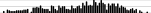nmissingdistinctInfoMeanGmd.05.10.25.50.75.90.95 5370529160.719.9828.4935.2247.9363.6774.4981.5485.56 lowest : 18.04199 18.41499 19.76500 20.29599 20.31200 , highest: 91.61896 91.81696 91.93396 92.73895 95.50995 death: Death at any time up to NDI date:31DEC94 nmissingdistinctInfoSumMeanGmd 537020.673560.66290.4477 sex nmissingdistinct 53702  Value female male Frequency 251 286 Proportion 0.467 0.533  hospdead: Death in Hospital nmissingdistinctInfoSumMeanGmd 537020.7032010.37430.4693 slos: Days from Study Entry to Discharge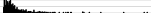nmissingdistinctInfoMeanGmd.05.10.25.50.75.90.95 5370850.99923.4422.24 4.0 5.0 9.015.027.047.468.2 lowest : 3 4 5 6 7 , highest: 145 164 202 236 241 d.time: Days of Follow-Up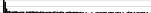nmissingdistinctInfoMeanGmd.05.10.25.50.75.90.95 53703401446.1566.1 4 6 16 182 72414211742 lowest : 3 4 5 6 7 , highest: 1977 1979 1982 2011 2022 dzgroup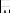nmissingdistinct 53703  Value ARF/MOSF w/Sepsis Coma MOSF w/Malig Frequency 391 60 86 Proportion 0.728 0.112 0.160  dzclass nmissingdistinct 53702  Value ARF/MOSF Coma Frequency 477 60 Proportion 0.888 0.112  num.co: number of comorbidities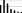nmissingdistinctInfoMeanGmd 537070.9261.5251.346 lowest : 0 1 2 3 4 , highest: 2 3 4 5 6  Value 0 1 2 3 4 5 6 Frequency 111 196 133 51 31 10 5 Proportion 0.207 0.365 0.248 0.095 0.058 0.019 0.009  edu: Years of Education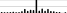nmissingdistinctInfoMeanGmd.05.10.25.50.75.90.95 411126220.95712.033.581 7 81012141617 lowest : 0 1 2 3 4 , highest: 17 18 19 20 22 income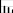nmissingdistinct 3352024  Value under$11k   $11-$25k   $25-$50k      >$50k Frequency 158 79 63 35 Proportion 0.472 0.236 0.188 0.104  scoma: SUPPORT Coma Score based on Glasgow D3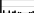nmissingdistinctInfoMeanGmd.05.10.25.50.75.90.95 5370110.82219.2427.87 0 0 0 0 37 55100 lowest : 0 9 26 37 41 , highest: 55 61 89 94 100  Value 0 9 26 37 41 44 55 61 89 94 100 Frequency 301 50 44 19 17 43 11 6 8 6 32 Proportion 0.561 0.093 0.082 0.035 0.032 0.080 0.020 0.011 0.015 0.011 0.060  charges: Hospital Charges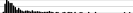nmissingdistinctInfoMeanGmd.05.10.25.50.75.90.95 5172051618665290079 11075 15180 27389 51079100904205562283411 lowest : 3448.0 4432.0 4574.0 5555.0 5849.0 , highest: 504659.5 538323.0 543761.0 706577.0 740010.0 totcst: Total RCC cost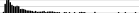nmissingdistinctInfoMeanGmd.05.10.25.50.75.90.95 4716647114636046195 6359 8449 15412 29308 57028108927141569  lowest : 0 2071.11 2522.45 3190.62 3325.35 highest: 269057 269131 338955 357919 390460 totmcst: Total micro-cost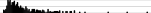nmissingdistinctInfoMeanGmd.05.10.25.50.75.90.95 33120632813902236200 6131 8283 14415 26323 54102 87495111920  lowest : 0 1561.62 2477.51 2626.27 3421.07 highest: 144234 154709 198047 234876 271467 avtisst: Average TISS, Days 3-25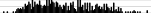nmissingdistinctInfoMeanGmd.05.10.25.50.75.90.95 5361205129.8314.1912.4614.5019.6228.0039.0047.1750.37  lowest : 4 5.66666 8 9 9.5 highest: 58.5 59 60 61 64 race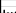nmissingdistinct 53525 lowest : white black asian other hispanic , highest: white black asian other hispanic  Value white black asian other hispanic Frequency 417 84 4 8 22 Proportion 0.779 0.157 0.007 0.015 0.041  meanbp: Mean Arterial Blood Pressure Day 3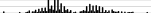nmissingdistinctInfoMeanGmd.05.10.25.50.75.90.95 5370109183.2835 41.8 49.0 59.0 73.0111.0124.4135.0 lowest : 0 20 27 30 32 , highest: 155 158 161 162 180 wblc: White Blood Cell Count Day 3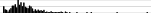nmissingdistinctInfoMeanGmd.05.10.25.50.75.90.95 5325241114.19.984 0.8999 4.5000 7.974912.398418.199225.189130.1873  lowest : 0.0499954 0.0699921 0.0999908 0.149994 0.199982 highest: 51.3984 58.1953 61.1953 79.3906 100 hrt: Heart Rate Day 3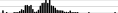nmissingdistinctInfoMeanGmd.05.10.25.50.75.90.95 53701110.99910538.59 51 60 75111126140155 lowest : 0 11 30 36 40 , highest: 189 193 199 232 300 resp: Respiration Rate Day 3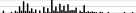nmissingdistinctInfoMeanGmd.05.10.25.50.75.90.95 5370450.99723.7212.65 8101224323940 lowest : 0 4 6 7 8 , highest: 48 49 52 60 64 temp: Temperature (celcius) Day 3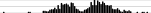nmissingdistinctInfoMeanGmd.05.10.25.50.75.90.95 5370610.99937.521.50535.5035.8036.4037.8038.5039.0939.50 lowest : 32.50000 34.00000 34.09375 34.89844 35.00000 , highest: 40.19531 40.59375 40.89844 41.00000 41.19531 pafi: PaO2/(.01*FiO2) Day 3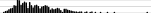nmissingdistinctInfoMeanGmd.05.10.25.50.75.90.95 500373571227.2125 86.99105.08137.88202.56290.00390.49433.31  lowest : 45 48 53.3281 54 55 highest: 574 595.125 640 680 869.375 alb: Serum Albumin Day 3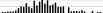nmissingdistinctInfoMeanGmd.05.10.25.50.75.90.95 346191340.9972.6680.72191.7001.9002.2252.6003.1003.4003.800 lowest : 1.099854 1.199951 1.299805 1.399902 1.500000 , highest: 4.099609 4.199219 4.500000 4.699219 4.799805 bili: Bilirubin Day 3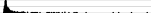n missing distinct Info Mean Gmd .05 .10 .25 386 151 88 0.997 2.678 3.507 0.3000 0.4000 0.6000 .50 .75 .90 .95 0.8999 2.0000 6.5996 13.1743   lowest : 0.0999908 0.199982 0.299988 0.399963 0.5 highest: 22.5977 30 31.5 35 39.2969 crea: Serum creatinine Day 3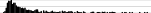nmissingdistinctInfoMeanGmd.05.10.25.50.75.90.95 5370840.9982.2321.9970.60000.70000.89991.39992.59965.23957.3197  lowest : 0.299988 0.399963 0.5 0.599976 0.699951 highest: 10.3984 10.5996 11.1992 11.5996 11.7988 sod: Serum sodium Day 3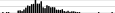nmissingdistinctInfoMeanGmd.05.10.25.50.75.90.95 5370380.997138.17.471129131134137142147150 lowest : 118 120 121 126 127 , highest: 156 157 158 168 175 ph: Serum pH (arterial) Day 3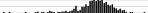nmissingdistinctInfoMeanGmd.05.10.25.50.75.90.95 50037490.9987.4160.087757.2707.3197.3807.4207.4707.5107.529 lowest : 6.959961 6.989258 7.069336 7.119141 7.129883 , highest: 7.559570 7.569336 7.589844 7.599609 7.659180 glucose: Glucose Day 3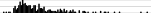nmissingdistinctInfoMeanGmd.05.10.25.50.75.90.95 2972401791167.792.13 76.0 89.0106.0141.0200.0292.4347.2 lowest : 30 42 52 55 68 , highest: 446 468 492 576 598 bun: BUN Day 3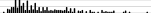nmissingdistinctInfoMeanGmd.05.10.25.50.75.90.95 304233100138.9131.12 8.00 11.00 16.75 30.00 56.00 79.70100.70 lowest : 1 3 4 5 6 , highest: 123 124 125 128 146 urine: Urine Output Day 3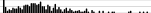nmissingdistinctInfoMeanGmd.05.10.25.50.75.90.95 303234262120951579 20.3 364.01156.51870.02795.04008.64817.5 lowest : 0 5 8 15 20 , highest: 6865 6920 7360 7560 7750 adlp: ADL Patient Day 3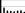nmissingdistinctInfoMeanGmd 10443380.8751.5772.152 lowest : 0 1 2 3 4 , highest: 3 4 5 6 7  Value 0 1 2 3 4 5 6 7 Frequency 51 19 7 6 4 7 8 2 Proportion 0.490 0.183 0.067 0.058 0.038 0.067 0.077 0.019  adls: ADL Surrogate Day 3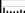nmissingdistinctInfoMeanGmd 39214580.8881.862.466 lowest : 0 1 2 3 4 , highest: 3 4 5 6 7  Value 0 1 2 3 4 5 6 7 Frequency 185 68 22 18 17 20 39 23 Proportion 0.472 0.173 0.056 0.046 0.043 0.051 0.099 0.059  sfdm2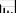nmissingdistinct 468695  lowest : no(M2 and SIP pres) adl>=4 (>=5 if sur) SIP>=30 Coma or Intub <2 mo. follow-up highest: no(M2 and SIP pres) adl>=4 (>=5 if sur) SIP>=30 Coma or Intub <2 mo. follow-up  Value no(M2 and SIP pres) adl>=4 (>=5 if sur) SIP>=30 Frequency 134 78 30 Proportion 0.286 0.167 0.064 Value Coma or Intub <2 mo. follow-up Frequency 5 221 Proportion 0.011 0.472  adlsc: Imputed ADL Calibrated to Surrogate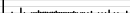nmissingdistinctInfoMeanGmd.05.10.25.50.75.90.95 53701440.9562.1192.3860.0000.0000.0001.8393.3756.0006.000  lowest : 0 0.494751 0.4948 1 1.16675 highest: 5.7832 6 6.33984 6.46582 7 B Code spar(ps=11) # Show patterns of missing data plot(naclus(support[acute,]))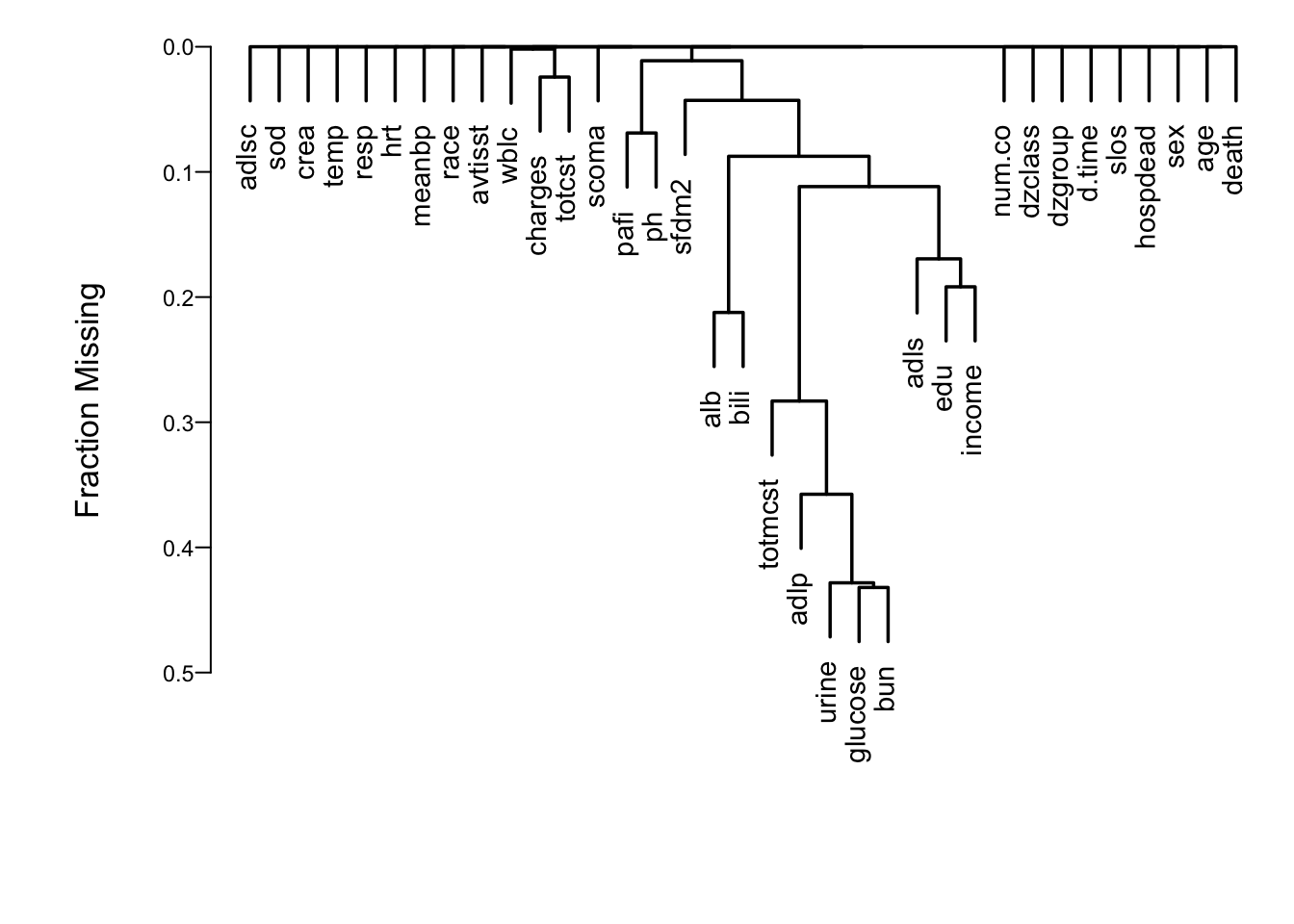Figure 19.1: Cluster analysis showing which predictors tend to be missing on the same patients Show associations between predictors using a general non-monotonic measure of dependence (Hoeffding $$D$$). C Code ac <- support[acute,] ac$dzgroup <- ac$dzgroup[drop=TRUE] # Remove unused levels vc <- varclus(~ age+sex+dzgroup+num.co+edu+income+scoma+race+ meanbp+wblc+hrt+resp+temp+pafi+alb+bili+crea+sod+ ph+glucose+bun+urine+adlsc, data=ac, sim='hoeffding') plot(vc)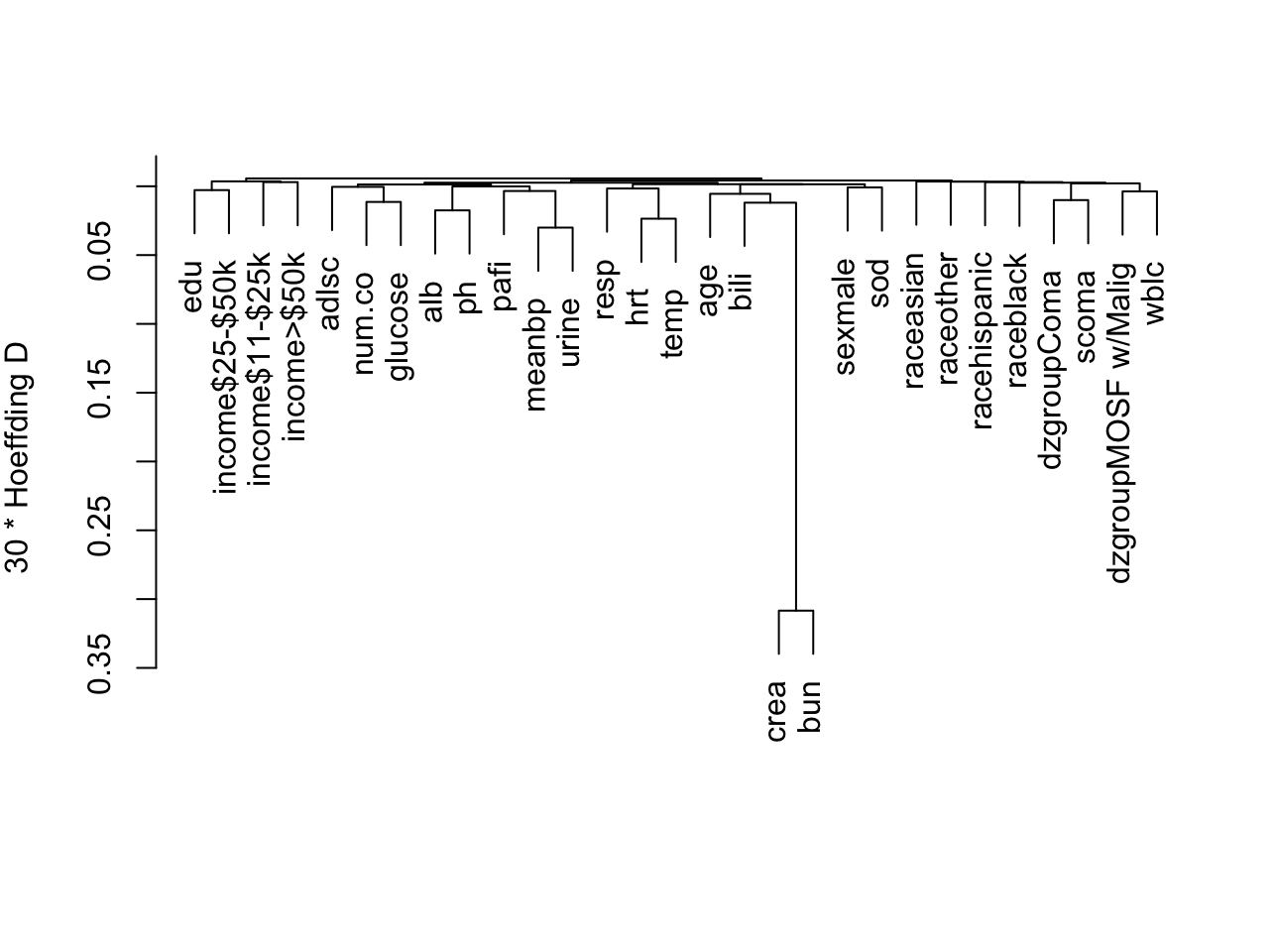Figure 19.2: Hierarchical clustering of potential predictors using Hoeffding $$D$$ as a similarity measure. Categorical predictors are automatically expanded into dummy variables. ## 19.2 Checking Adequacy of Log-Normal Accelerated Failure Time Model Code dd <- datadist(ac) # describe distributions of variables to rms options(datadist='dd') # Generate right-censored survival time variable ac <- upData(ac, print=FALSE, years = d.time/365.25, units = c(years = 'Year'), S = Surv(years, death)) # Show normal inverse Kaplan-Meier estimates # stratified by dzgroup survplot(npsurv(S ~ dzgroup, data=ac), conf='none', fun=qnorm,logt=TRUE)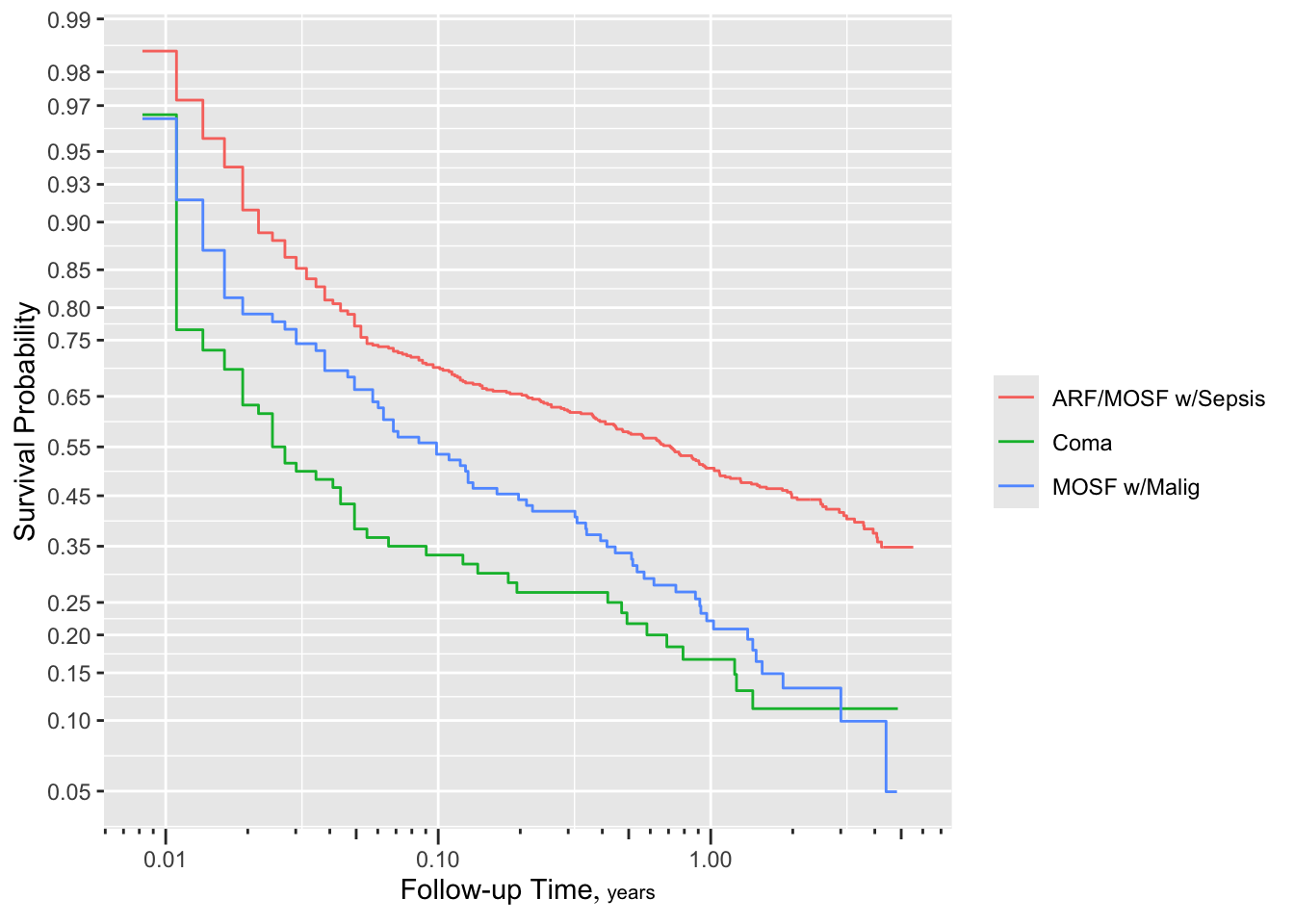Figure 19.3: $$\Phi^{-1}(S_{ ext{KM}}(t))$$ stratified by dzgroup. Linearity and semi-parallelism indicate a reasonable fit to the log-normal accelerated failure time model with respect to one predictor. More stringent assessment of log-normal assumptions: check distribution of residuals from an adjusted model: D Code spar(mfrow=c(2,2), ps=8, top=1, lwd=1) f <- psm(S ~ dzgroup + rcs(age,5) + rcs(meanbp,5), dist='lognormal', y=TRUE, data=ac) r <- resid(f) with(ac, { survplot(r, dzgroup, label.curve=FALSE) survplot(r, age, label.curve=FALSE) survplot(r, meanbp, label.curve=FALSE) survplot(r, runif(length(age)), label.curve=FALSE) } )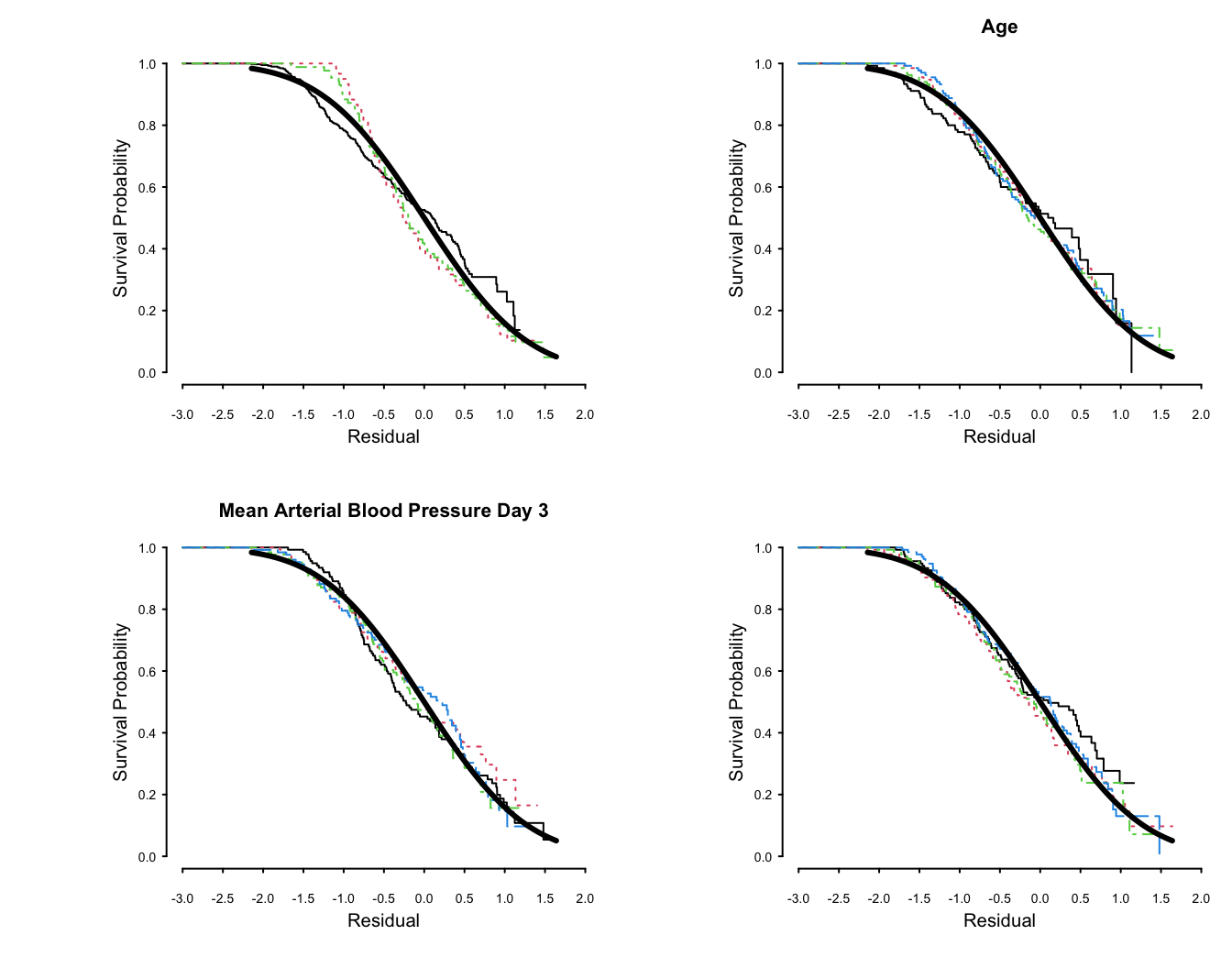Figure 19.4: Kaplan-Meier estimates of distributions of normalized, right-censored residuals from the fitted log-normal survival model. Residuals are stratified by important variables in the model (by quartiles of continuous variables), plus a random variable to depict the natural variability (in the lower right plot). Theoretical standard Gaussian distributions of residuals are shown with a thick solid line. The upper left plot is with respect to disease group. The fit for dzgroup is not great but overall fit is good. Remove from consideration predictors that are missing in $$> 0.2$$ of the patients. Many of these were only collected for the second phase of SUPPORT. Of those variables to be included in the model, find which ones have enough potential predictive power to justify allowing for nonlinear relationships or multiple categories, which spend more d.f. For each variable compute Spearman $$\rho^2$$ based on multiple linear regression of rank($$x$$), rank($$x$$)$$^2$$ and the survival time, truncating survival time at the shortest follow-up for survivors (356 days). This rids the data of censoring but creates many ties at 356 days. Code spar(top=1, ps=10, rt=3) ac <- upData(ac, print=FALSE, shortest.follow.up = min(d.time[death==0], na.rm=TRUE), d.timet = pmin(d.time, shortest.follow.up)) w <- spearman2(d.timet ~ age + num.co + scoma + meanbp + hrt + resp + temp + crea + sod + adlsc + wblc + pafi + ph + dzgroup + race, p=2, data=ac) plot(w, main='')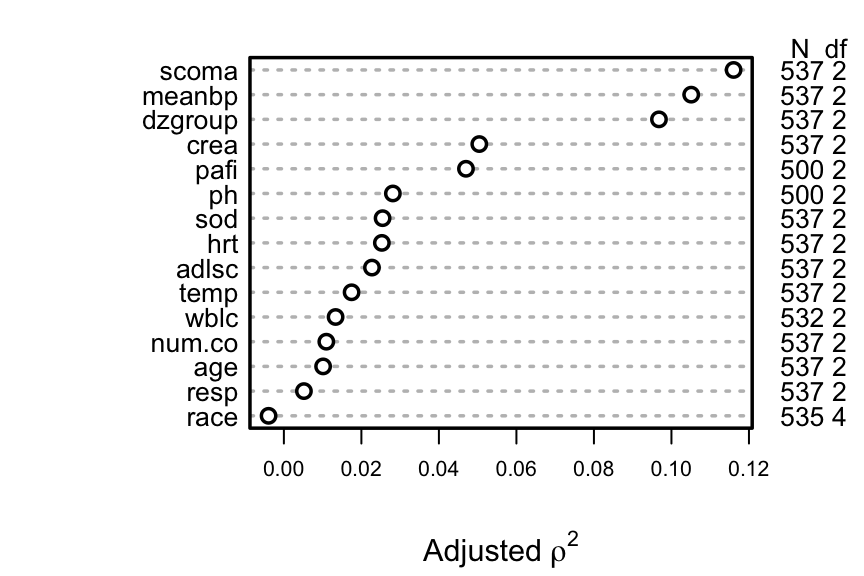Figure 19.5: Generalized Spearman $$\rho^2$$ rank correlation between predictors and truncated survival time A better approach is to use the complete information in the failure and censoring times by computing Somers’ $$D_{xy}$$ rank correlation allowing for censoring. E Code spar(top=1, ps=10, rt=3) w <- rcorrcens(S ~ age + num.co + scoma + meanbp + hrt + resp + temp + crea + sod + adlsc + wblc + pafi + ph + dzgroup + race, data=ac) plot(w, main='')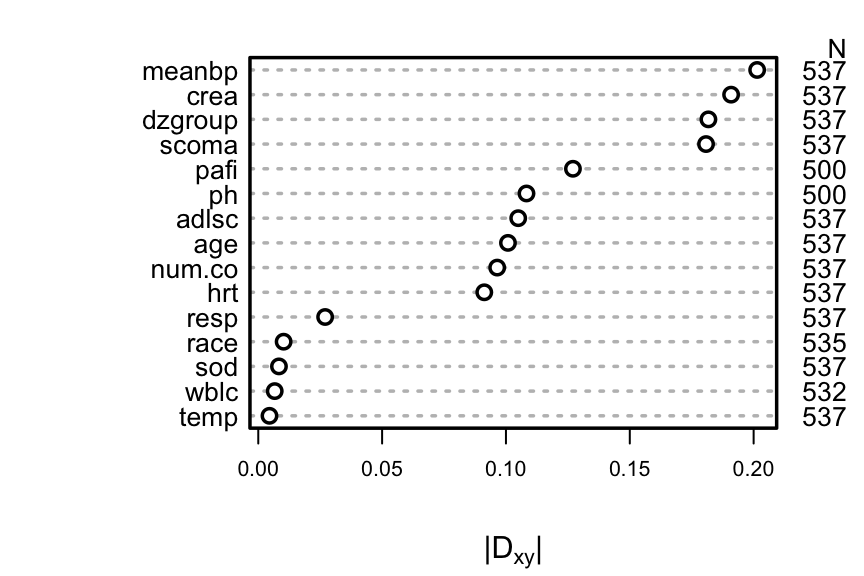Figure 19.6: Somers’ $$D_{xy}$$ rank correlation between predictors and original survival time. For dzgroup or race, the correlation coefficient is the maximum correlation from using a dummy variable to represent the most frequent or one to represent the second most frequent category. Code # Compute number of missing values per variable sapply(ac[.q(age,num.co,scoma,meanbp,hrt,resp,temp,crea,sod,adlsc, wblc,pafi,ph)], function(x) sum(is.na(x)))  age num.co scoma meanbp hrt resp temp crea sod adlsc wblc 0 0 0 0 0 0 0 0 0 0 5 pafi ph 37 37  Code # Can also do naplot(naclus(support[acute,])) # Can also use the Hmisc naclus and naplot functions to do this # Impute missing values with normal or modal values ac <- upData(ac, print=FALSE, wblc.i = impute(wblc, 9), pafi.i = impute(pafi, 333.3), ph.i = impute(ph, 7.4), race2 = ifelse(is.na(race), 'white', ifelse(race != 'white', 'other', 'white')) ) dd <- datadist(ac) Do a formal redundancy analysis using more than pairwise associations, and allow for non-monotonic transformations in predicting each predictor from all other predictors. This analysis requires missing values to be imputed so as to not greatly reduce the sample size. F Code redun(~ crea + age + sex + dzgroup + num.co + scoma + adlsc + race2 + meanbp + hrt + resp + temp + sod + wblc.i + pafi.i + ph.i, data=ac, nk=4)  Redundancy Analysis redun(formula = ~crea + age + sex + dzgroup + num.co + scoma + adlsc + race2 + meanbp + hrt + resp + temp + sod + wblc.i + pafi.i + ph.i, data = ac, nk = 4) n: 537 p: 16 nk: 4 Number of NAs: 0 Transformation of target variables forced to be linear R-squared cutoff: 0.9 Type: ordinary R^2 with which each variable can be predicted from all other variables: crea age sex dzgroup num.co scoma adlsc race2 meanbp hrt 0.133 0.246 0.132 0.451 0.147 0.418 0.153 0.151 0.178 0.258 resp temp sod wblc.i pafi.i ph.i 0.131 0.197 0.135 0.093 0.143 0.171 No redundant variables Better approach to gauging predictive potential and allocating d.f.: • Allow all continuous variables to have a the maximum number of knots entertained, in a log-normal survival model • Must use imputation to avoid losing data • Fit a “saturated” main effects model • Makes full use of censored data • Had to limit to 4 knots, force scoma to be linear, and omit ph.i to avoid singularity G Code k <- 4 f <- psm(S ~ rcs(age,k)+sex+dzgroup+pol(num.co,2)+scoma+ pol(adlsc,2)+race+rcs(meanbp,k)+rcs(hrt,k)+rcs(resp,k)+ rcs(temp,k)+rcs(crea,k)+rcs(sod,k)+rcs(wblc.i,k)+ rcs(pafi.i,k), dist='lognormal', data=ac) plot(anova(f))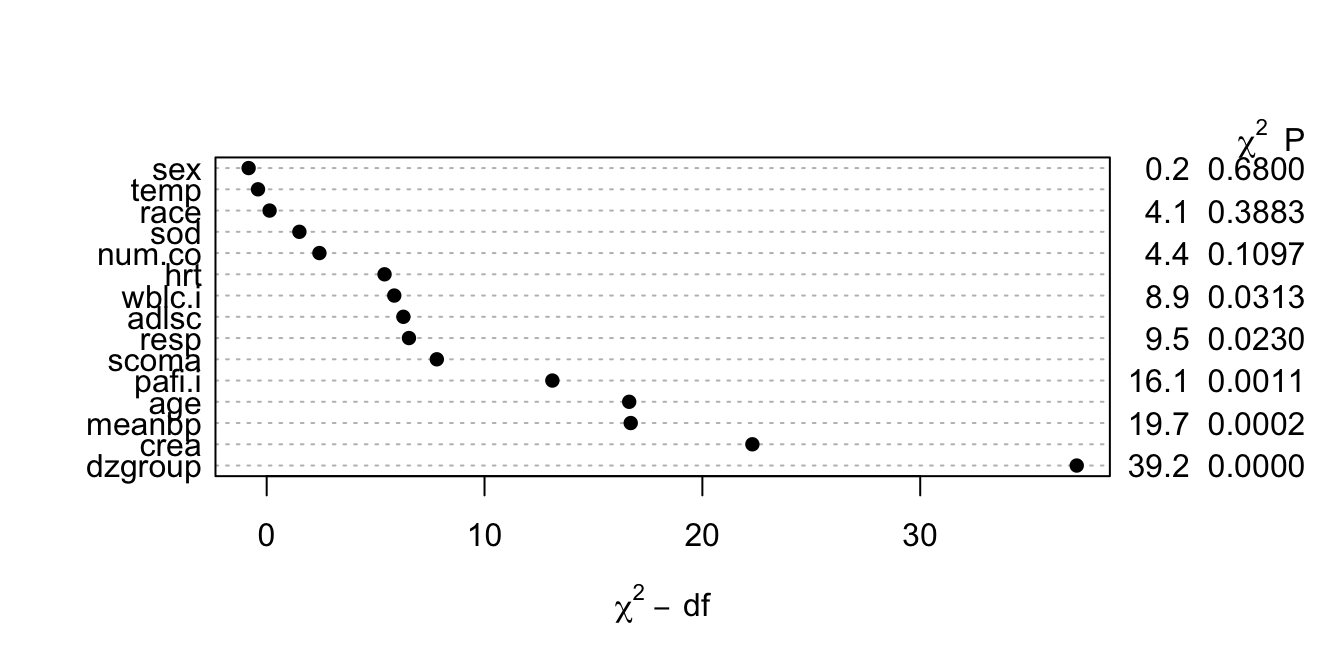Figure 19.7: Partial $$\chi^{2}$$ statistics for association of each predictor with response from saturated main effects model, penalized for d.f. • This figure properly blinds the analyst to the form of effects (tests of linearity). • Fit a log-normal survival model with number of parameters corresponding to nonlinear effects determined from Figure 19.7. For the most promising predictors, five knots can be allocated, as there are fewer singularity problems once less promising predictors are simplified. HI Note: Since the audio was recorded, a bug in psm was fixed on 2017-03-12. Discrimination indexes shown in the table below are correct but the audio is incorrect for $$g$$ and $$g_{r}$$. Code f <- psm(S ~ rcs(age,5) + sex + dzgroup + num.co + scoma + pol(adlsc,2) + race2 + rcs(meanbp,5) + rcs(hrt,3) + rcs(resp,3) + temp + rcs(crea,4) + sod + rcs(wblc.i,3) + rcs(pafi.i,4), dist='lognormal', data=ac) f Parametric Survival Model: Log Normal Distribution  psm(formula = S ~ rcs(age, 5) + sex + dzgroup + num.co + scoma + pol(adlsc, 2) + race2 + rcs(meanbp, 5) + rcs(hrt, 3) + rcs(resp, 3) + temp + rcs(crea, 4) + sod + rcs(wblc.i, 3) + rcs(pafi.i, 4), data = ac, dist = "lognormal")  Model Likelihood Ratio Test Discrimination Indexes Obs 537 LR χ2 236.83 R2 0.594 Events 356 d.f. 30 R230,537 0.320 σ 2.230782 Pr(>χ2) <0.0001 R230,356 0.441 Dxy 0.485 β S.E. Wald Z Pr(>|Z|) (Intercept) -5.3904 3.7603 -1.43 0.1517 age -0.0148 0.0309 -0.48 0.6322 age’ -0.0412 0.1078 -0.38 0.7024 age’’ 0.1670 0.5594 0.30 0.7653 age’’’ -0.2099 1.3707 -0.15 0.8783 sex=male -0.0737 0.2181 -0.34 0.7354 dzgroup=Coma -2.0676 0.4062 -5.09 <0.0001 dzgroup=MOSF w/Malig -1.4664 0.3112 -4.71 <0.0001 num.co -0.1917 0.0858 -2.23 0.0255 scoma -0.0142 0.0044 -3.25 0.0011 adlsc -0.3735 0.1520 -2.46 0.0140 adlsc2 0.0442 0.0243 1.82 0.0691 race2=white -0.2979 0.2658 -1.12 0.2624 meanbp 0.0702 0.0210 3.34 0.0008 meanbp’ -0.3080 0.2261 -1.36 0.1732 meanbp’’ 0.8438 0.8556 0.99 0.3241 meanbp’’’ -0.5715 0.7707 -0.74 0.4584 hrt -0.0171 0.0069 -2.46 0.0140 hrt’ 0.0064 0.0063 1.02 0.3090 resp 0.0454 0.0230 1.97 0.0483 resp’ -0.0851 0.0291 -2.93 0.0034 temp 0.0523 0.0834 0.63 0.5308 crea -0.4585 0.6727 -0.68 0.4955 crea’ -11.5176 19.0027 -0.61 0.5444 crea’’ 21.9840 31.0113 0.71 0.4784 sod 0.0044 0.0157 0.28 0.7792 wblc.i 0.0746 0.0331 2.25 0.0242 wblc.i’ -0.0880 0.0377 -2.34 0.0195 pafi.i 0.0169 0.0055 3.07 0.0021 pafi.i’ -0.0569 0.0239 -2.38 0.0173 pafi.i’’ 0.1088 0.0482 2.26 0.0239 Log(scale) 0.8024 0.0401 19.99 <0.0001 Code a <- anova(f) ## 19.3 Summarizing the Fitted Model • Plot the shape of the effect of each predictor on log survival time. • All effects centered: can be placed on common scale • Wald $$\chi^2$$ statistics, penalized for d.f., plotted in descending order J Code ggplot(Predict(f, ref.zero=TRUE), vnames='names', sepdiscrete='vertical', anova=a)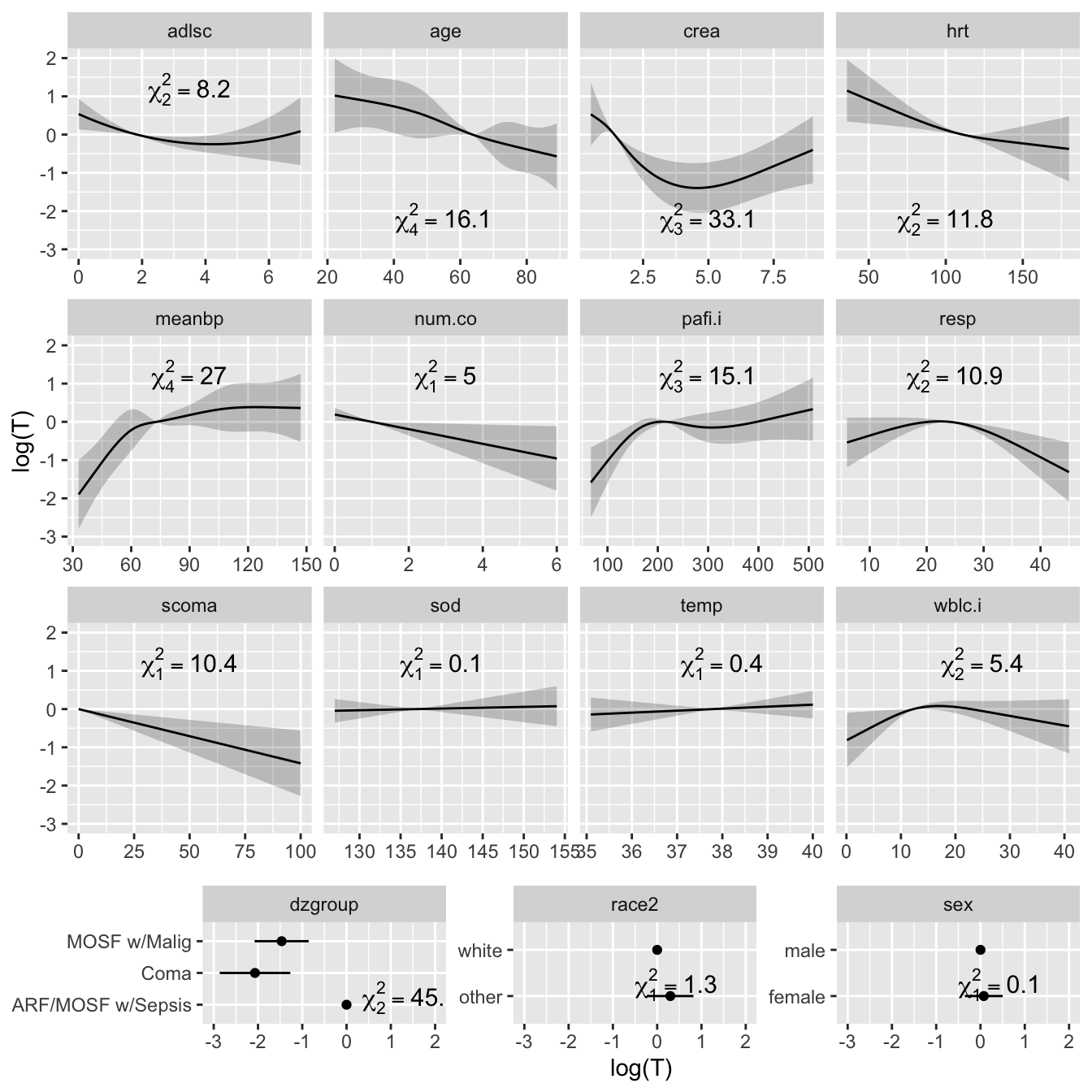Figure 19.8: Effect of each predictor on log survival time. Predicted values have been centered so that predictions at predictor reference values are zero. Pointwise 0.95 confidence bands are also shown. As all $$Y$$-axes have the same scale, it is easy to see which predictors are strongest. K Code a  χ2 d.f. P Wald Statistics for S age 15.99 4 0.0030 Nonlinear 0.23 3 0.9722 sex 0.11 1 0.7354 dzgroup 45.69 2 <0.0001 num.co 4.99 1 0.0255 scoma 10.58 1 0.0011 adlsc 8.28 2 0.0159 Nonlinear 3.31 1 0.0691 race2 1.26 1 0.2624 meanbp 27.62 4 <0.0001 Nonlinear 10.51 3 0.0147 hrt 11.83 2 0.0027 Nonlinear 1.04 1 0.3090 resp 11.10 2 0.0039 Nonlinear 8.56 1 0.0034 temp 0.39 1 0.5308 crea 33.63 3 <0.0001 Nonlinear 21.27 2 <0.0001 sod 0.08 1 0.7792 wblc.i 5.47 2 0.0649 Nonlinear 5.46 1 0.0195 pafi.i 15.37 3 0.0015 Nonlinear 6.97 2 0.0307 TOTAL NONLINEAR 60.48 14 <0.0001 TOTAL 261.47 30 <0.0001 Code plot(a)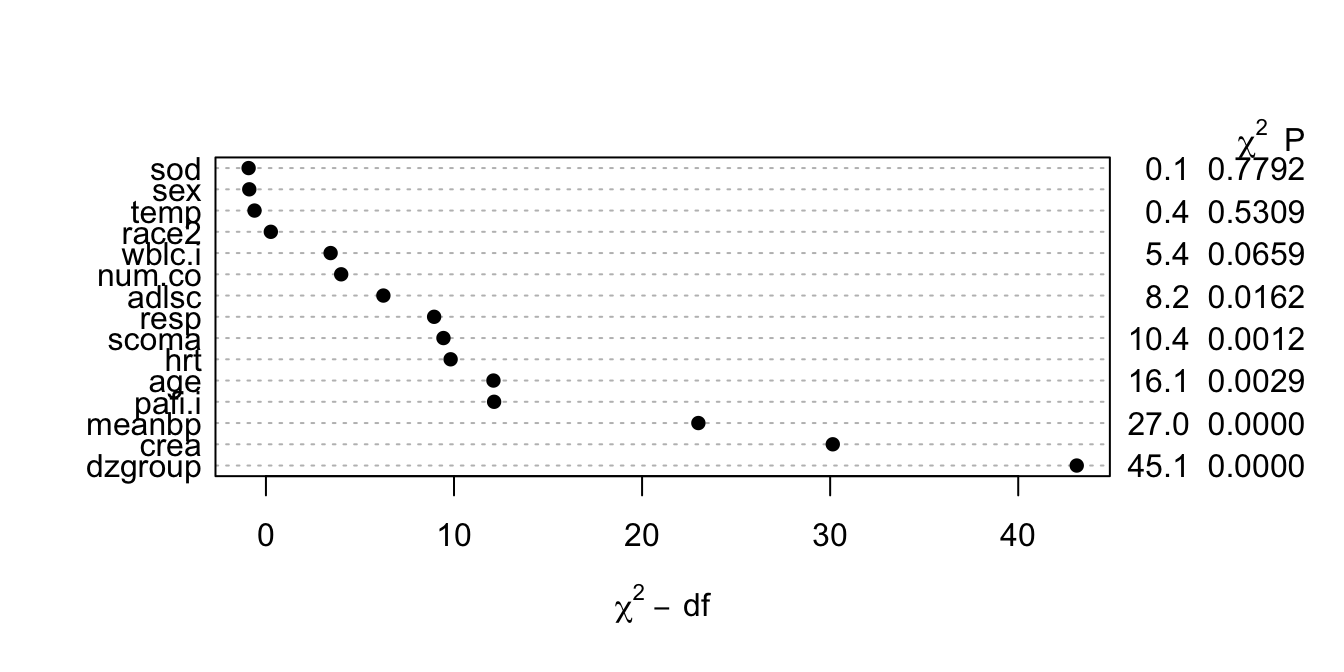Figure 19.9: Contribution of variables in predicting survival time in log-normal model L Code spar(top=1, ps=11) options(digits=3) plot(summary(f), log=TRUE, main='')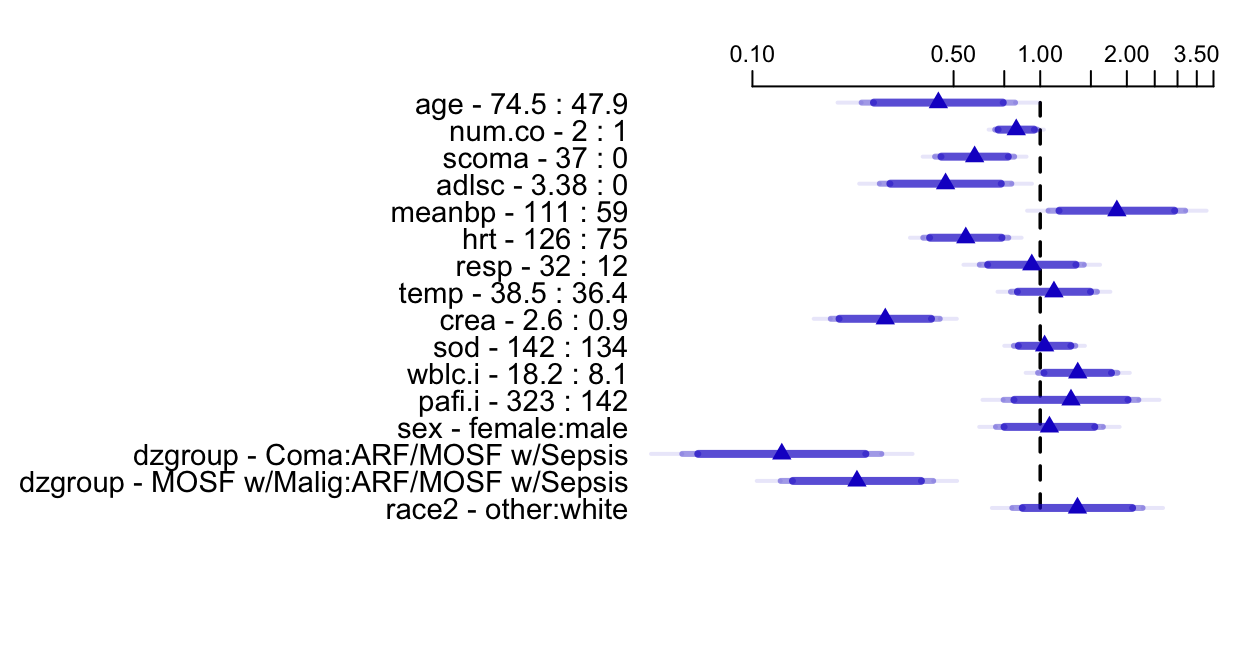Figure 19.10: Estimated survival time ratios for default settings of predictors. For example, when age changes from its lower quartile to the upper quartile (47.9y to 74.5y), median survival time decreases by more than half. Different shaded areas of bars indicate different confidence levels (0.9, 0.95, 0.99). ## 19.4 Internal Validation of the Fitted Model Using the Bootstrap Validate indexes describing the fitted model. Code # First add data to model fit so bootstrap can re-sample # from the data g <- update(f, x=TRUE, y=TRUE) set.seed(717) html(validate(g, B=120, dxy=TRUE)) Index Original Sample Training Sample Test Sample Optimism Corrected Index $$n$$ $$D_{xy}$$ 0.4852 0.5084 0.4593 0.0491 0.4362 120 $$R^{2}$$ 0.594 0.6587 0.5375 0.1211 0.4729 120 Intercept 0 0 -0.0313 0.0313 -0.0313 120 Slope 1 1 0.9049 0.0951 0.9049 120 $$D$$ 0.4788 0.5508 0.4229 0.1279 0.3509 120 $$U$$ -0.0041 -0.0041 -0.0098 0.0057 -0.0098 120 $$Q$$ 0.4829 0.5549 0.4328 0.1222 0.3607 120 $$g$$ 1.9593 2.0421 1.8643 0.1778 1.7816 120 • From $$D_{xy}$$ and $$R^2$$ there is a moderate amount of overfitting. • Slope shrinkage factor (0.90) is not troublesome • Almost unbiased estimate of future predictive discrimination on similar patients is the corrected $$D_{xy}$$ of 0.43. M Validate predicted 1-year survival probabilities. Use a smooth approach that does not require binning and use less precise Kaplan-Meier estimates obtained by stratifying patients by the predicted probability, with at least 60 patients per group. N Code set.seed(717) cal <- calibrate(g, u=1, B=120) plot(cal, subtitles=FALSE) cal <- calibrate(g, cmethod='KM', u=1, m=60, B=120, pr=FALSE) plot(cal, add=TRUE)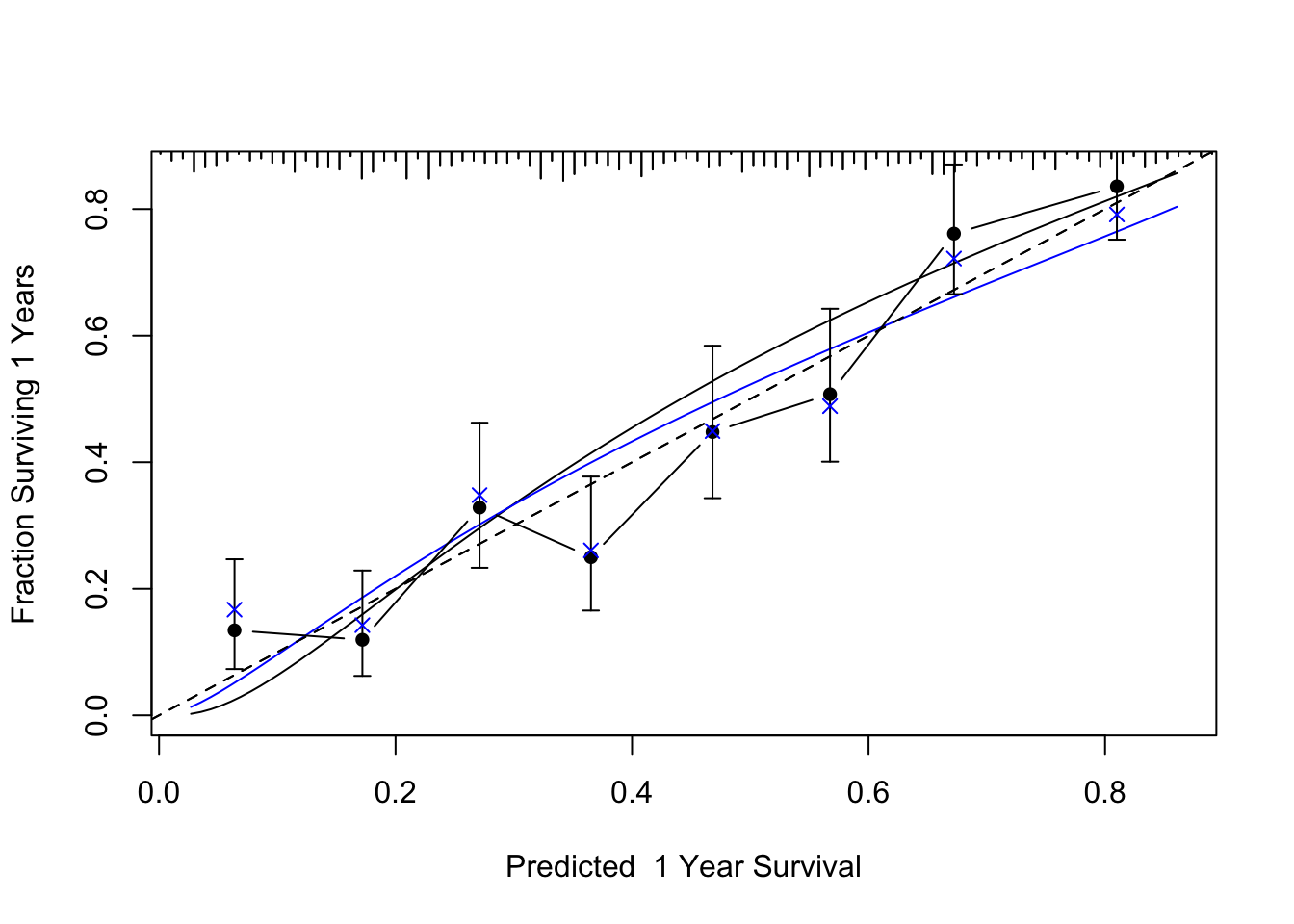Figure 19.11: Bootstrap validation of calibration curve. Dots represent apparent calibration accuracy; $$\times$$ are bootstrap estimates corrected for overfitting, based on binning predicted survival probabilities and and computing Kaplan-Meier estimates. Black curve is the estimated observed relationship using hare and the blue curve is the overfitting-corrected hare estimate. The gray-scale line depicts the ideal relationship. ## 19.5 Approximating the Full Model The fitted log-normal model is perhaps too complex for routine use and for routine data collection. Let us develop a simplified model that can predict the predicted values of the full model with high accuracy ($$R^{2} = 0.96$$). The simplification is done using a fast backward stepdown against the full model predicted values. Code Z <- predict(f) # X*beta hat a <- ols(Z ~ rcs(age,5)+sex+dzgroup+num.co+ scoma+pol(adlsc,2)+race2+ rcs(meanbp,5)+rcs(hrt,3)+rcs(resp,3)+ temp+rcs(crea,4)+sod+rcs(wblc.i,3)+ rcs(pafi.i,4), sigma=1, data=ac) # sigma=1 is used to prevent sigma hat from being zero when # R2=1.0 since we start out by approximating Z with all # component variables fastbw(a, aics=10000) # fast backward stepdown  Deleted Chi-Sq d.f. P Residual d.f. P AIC R2 sod 0.43 1 0.512 0.43 1 0.5117 -1.57 1.000 sex 0.57 1 0.451 1.00 2 0.6073 -3.00 0.999 temp 2.20 1 0.138 3.20 3 0.3621 -2.80 0.998 race2 6.81 1 0.009 10.01 4 0.0402 2.01 0.994 wblc.i 29.52 2 0.000 39.53 6 0.0000 27.53 0.976 num.co 30.84 1 0.000 70.36 7 0.0000 56.36 0.957 resp 54.18 2 0.000 124.55 9 0.0000 106.55 0.924 adlsc 52.46 2 0.000 177.00 11 0.0000 155.00 0.892 pafi.i 66.78 3 0.000 243.79 14 0.0000 215.79 0.851 scoma 78.07 1 0.000 321.86 15 0.0000 291.86 0.803 hrt 83.17 2 0.000 405.02 17 0.0000 371.02 0.752 age 68.08 4 0.000 473.10 21 0.0000 431.10 0.710 crea 314.47 3 0.000 787.57 24 0.0000 739.57 0.517 meanbp 403.04 4 0.000 1190.61 28 0.0000 1134.61 0.270 dzgroup 441.28 2 0.000 1631.89 30 0.0000 1571.89 0.000 Approximate Estimates after Deleting Factors Coef S.E. Wald Z P [1,] -0.5928 0.04315 -13.74 0 Factors in Final Model None Code f.approx <- ols(Z ~ dzgroup + rcs(meanbp,5) + rcs(crea,4) + rcs(age,5) + rcs(hrt,3) + scoma + rcs(pafi.i,4) + pol(adlsc,2)+ rcs(resp,3), x=TRUE, data=ac) f.approx$stats
         n Model L.R.       d.f.         R2          g      Sigma
537.000   1688.225     23.000      0.957      1.915      0.370 
• Estimate variance-covariance matrix of the coefficients of reduced model
• This covariance matrix does not include the scale parameter
O
Code
V <- vcov(f, regcoef.only=TRUE)     # var(full model)
X <- cbind(Intercept=1, g$x) # full model design x <- cbind(Intercept=1, f.approx$x) # approx. model design
w <- solve(t(x) %*% x, t(x)) %*% X  # contrast matrix
v <- w %*% V %*% t(w)

Compare variance estimates (diagonals of v) with variance estimates from a reduced model that is fitted against the actual outcomes.

Code
f.sub <- psm(S ~ dzgroup + rcs(meanbp,5) + rcs(crea,4) + rcs(age,5) +
rcs(hrt,3) + scoma + rcs(pafi.i,4) + pol(adlsc,2)+
rcs(resp,3), dist='lognormal', data=ac)

r <- diag(v)/diag(vcov(f.sub,regcoef.only=TRUE))
r[c(which.min(r), which.max(r))]
 hrt'   age
0.976 0.982 

P
Code
f.approx\$var <- v
anova(f.approx, test='Chisq', ss=FALSE)
 χ2 d.f. P Wald Statistics for Z dzgroup 55.94 2 <0.0001 meanbp 29.87 4 <0.0001 Nonlinear 9.84 3 0.0200 crea 39.04 3 <0.0001 Nonlinear 24.37 2 <0.0001 age 18.12 4 0.0012 Nonlinear 0.34 3 0.9517 hrt 9.87 2 0.0072 Nonlinear 0.40 1 0.5289 scoma 9.85 1 0.0017 pafi.i 14.01 3 0.0029 Nonlinear 6.66 2 0.0357 adlsc 9.71 2 0.0078 Nonlinear 2.87 1 0.0904 resp 9.65 2 0.0080 Nonlinear 7.13 1 0.0076 TOTAL NONLINEAR 58.08 13 <0.0001 TOTAL 252.32 23 <0.0001

Equation for simplified model:

Code
# Typeset mathematical form of approximate model
latex(f.approx)
$\text{E}(\text{Z}) = X\beta,~~\text{where}$ $\begin{array} \lefteqn{X\hat{\beta}=}\\ & & -2.51 \\ & & -1.94[\text{Coma}]-1.75[\text{MOSF\ w/Malig}] \\ & & + 0.068 \text{meanbp}-3.08\!\times\!10^{-5}(\text{meanbp}-41.8)_{+}^{3}+7.9\!\times\!10^{-5 }(\text{meanbp}-61)_{+}^{3} \\ & & -4.91\!\times\!10^{-5}(\text{meanbp}-73)_{+}^{3}+2.61\!\times\!10^{-6 }(\text{meanbp}-109)_{+}^{3}-1.7\!\times\!10^{-6 }(\text{meanbp}-135)_{+}^{3} \\ & & -0.553\text{crea}-0.229(\text{crea}-0.6)_{+}^{3}+0.45 (\text{crea}-1.1)_{+}^{3}-0.233(\text{crea}-1.94)_{+}^{3} \\ & & +0.0131(\text{crea}-7.32)_{+}^{3} \\ & & -0.0165 \text{age}-1.13\!\times\!10^{-5}(\text{age}-28.5)_{+}^{3}+4.05\!\times\!10^{-5 }(\text{age}-49.5)_{+}^{3} \\ & & -2.15\!\times\!10^{-5}(\text{age}-63.7)_{+}^{3}-2.68\!\times\!10^{-5}(\text{age}-72.7)_{+}^{3}+1.9\!\times\!10^{-5 }(\text{age}-85.6)_{+}^{3} \\ & & -0.0136 \text{hrt}+6.09\!\times\!10^{-7 }(\text{hrt}-60)_{+}^{3}-1.68\!\times\!10^{-6}(\text{hrt}-111)_{+}^{3}+1.07\!\times\!10^{-6 }(\text{hrt}-140)_{+}^{3} \\ & & -0.0135\:\text{scoma} \\ & & + 0.0161 \text{pafi.i}-4.77\!\times\!10^{-7}(\text{pafi.i}-88)_{+}^{3}+9.11\!\times\!10^{-7 }(\text{pafi.i}-167)_{+}^{3} \\ & & -5.02\!\times\!10^{-7}(\text{pafi.i}-276)_{+}^{3}+6.76\!\times\!10^{-8 }(\text{pafi.i}-426)_{+}^{3} -0.369\:\text{adlsc}+0.0409\:\text{adlsc}^{2} \\ & & + 0.0394 \text{resp}-9.11\!\times\!10^{-5}(\text{resp}-10)_{+}^{3}+0.000176 (\text{resp}-24)_{+}^{3}-8.5\!\times\!10^{-5 }(\text{resp}-39)_{+}^{3} \\ \end{array}$

$[c]=1~\text{if subject is in group}~c,~0~\text{otherwise}$$(x)_{+}=x~\text{if}~x > 0,~0~\text{otherwise}$

Nomogram for predicting median and mean survival time, based on approximate model:

Code
# Derive R functions that express mean and quantiles
# of survival time for specific linear predictors
# analytically
expected.surv <- Mean(f)
quantile.surv <- Quantile(f)
expected.surv
function (lp = NULL, parms = 0.802352037606488)
{
names(parms) <- NULL
exp(lp + exp(2 * parms)/2)
}
<environment: namespace:rms>
Code
quantile.surv
function (q = 0.5, lp = NULL, parms = 0.802352037606488)
{
names(parms) <- NULL
f <- function(lp, q, parms) lp + exp(parms) * qnorm(q)
names(q) <- format(q)
drop(exp(outer(lp, q, FUN = f, parms = parms)))
}
<environment: namespace:rms>
Code
median.surv   <- function(x) quantile.surv(lp=x)
Code
spar(ps=10)
# Improve variable labels for the nomogram
f.approx <- Newlabels(f.approx, c('Disease Group','Mean Arterial BP',
'Creatinine','Age','Heart Rate','SUPPORT Coma Score',
nom <-
nomogram(f.approx,
pafi.i=c(0, 50, 100, 200, 300, 500, 600, 700, 800, 900),
fun=list('Median Survival Time'=median.surv,
'Mean Survival Time'  =expected.surv),
fun.at=c(.1,.25,.5,1,2,5,10,20,40))
plot(nom, cex.var=1, cex.axis=.75, lmgp=.25)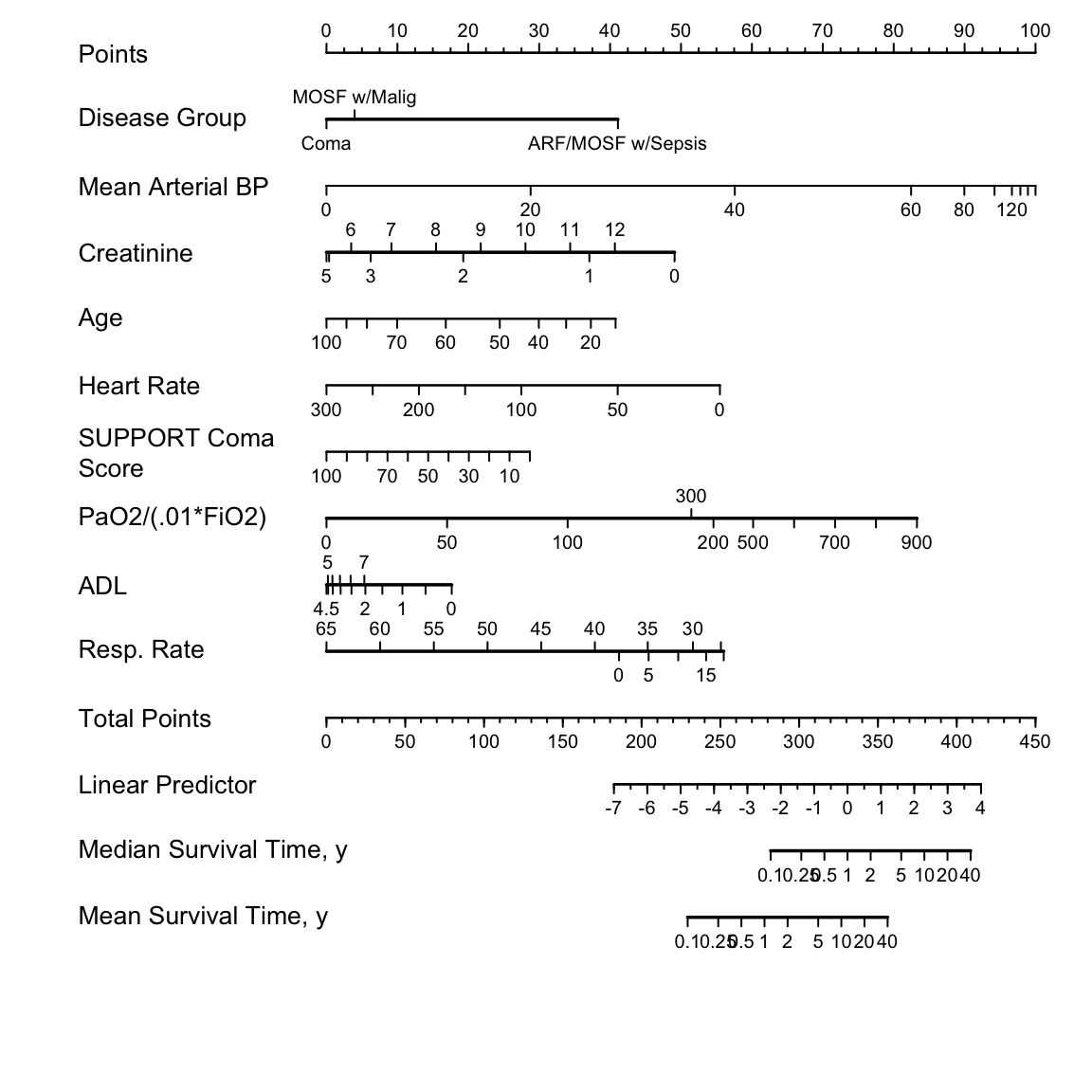Figure 19.12: Nomogram for predicting median and mean survival time, based on approximation of full model
R packages and functions used. All packages are available on CRAN.
Packages Purpose Functions
Hmisc Miscellaneous functions describe,ecdf,naclus,varclus,llist,spearman2,impute,latex
rms Modeling datadist,psm,rcs,ols,fastbw
Model presentation survplot,Newlabels,Function,Mean,Quantile,nomogram
Model validation validate,calibrate